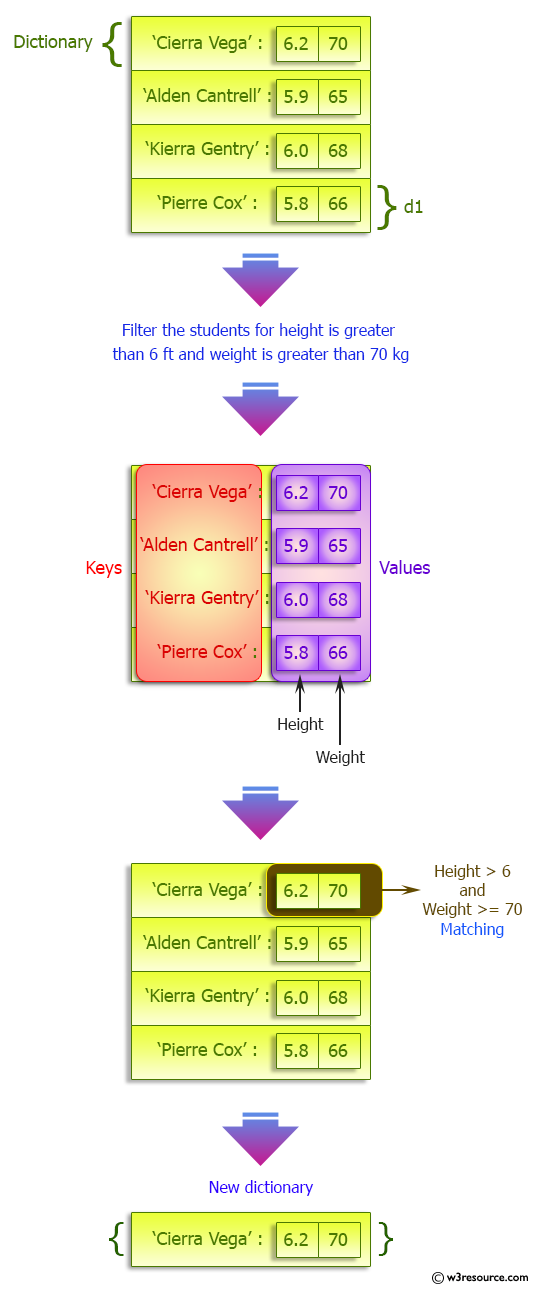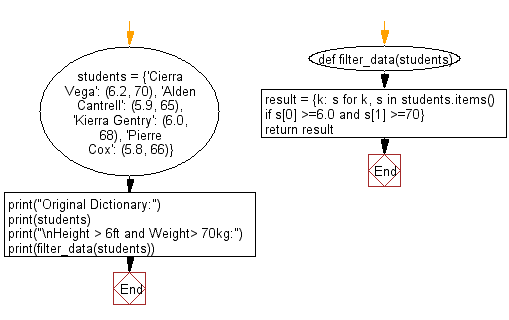﻿ Python: Filter the height and width of students which are stored in a dictionary - w3resource# Python: Filter the height and width of students which are stored in a dictionary

## Python dictionary: Exercise-44 with Solution

Write a Python program to filter the height and width of students, which are stored in a dictionary.

Sample Solution:

Python Code:

``````def filter_data(students):
result = {k: s for k, s in students.items() if s >=6.0 and s >=70}
return result

students = {'Cierra Vega': (6.2, 70), 'Alden Cantrell': (5.9, 65), 'Kierra Gentry': (6.0, 68), 'Pierre Cox': (5.8, 66)}
print("Original Dictionary:")
print(students)
print("\nHeight > 6ft and Weight> 70kg:")
print(filter_data(students))
```
```

Sample Output:

```Original Dictionary:
{'Cierra Vega': (6.2, 70), 'Alden Cantrell': (5.9, 65), 'Kierra Gentry': (6.0, 68), 'Pierre Cox': (5.8, 66)}

Height > 6ft and Weight> 70kg:
{'Cierra Vega': (6.2, 70)}
```

Pictorial Presentation:Flowchart:## Visualize Python code execution:

The following tool visualize what the computer is doing step-by-step as it executes the said program:

Python Code Editor:

Have another way to solve this solution? Contribute your code (and comments) through Disqus.

What is the difficulty level of this exercise?

Test your Programming skills with w3resource's quiz.

﻿

## Python: Tips of the Day

Invokes the provided function after ms milliseconds:

Example:

```from time import sleep

def tips_delay(fn, ms, *args):
sleep(ms / 1000)
return fn(*args)
print(tips_delay(
lambda x: print(x),
1000,
'w3r'
))
```

Output:

```w3r
None
```